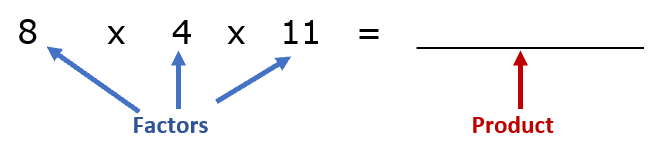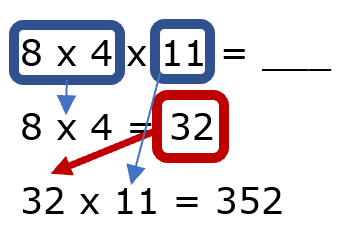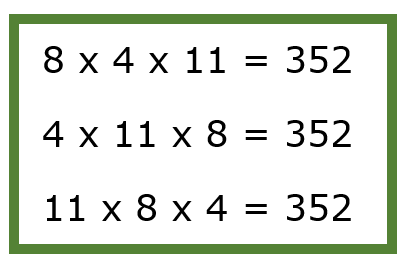Home > Math Topics > Basic Operations >

## Commutative Property Worksheets

#### Operations - 1.OA.3, 3.OA.5

The commutative property applies to the mathematical operations of both addition and subtraction. The property just tells us that it makes no difference to the answer as to what order the terms in problems appear. In addition, there are three parts to problem: the addends, the equal sign, and the sum. The order of the addends does not matter at all. For instance, 4 + 3 = 7 is the same thing as 3 + 4 = 7. Multiplication is remarkably similar to this, there four parts in a multiplication exercise: the product, equal sign, multiplicand, and multiplier. In this case the multiplicand and multiplier do not matter at all, you will still get the same product. For instance: 7 x 3 = 21 is the same as 3 x 7 = 21. Please note that this does not apply to all math operations as this does not work for subtraction or division. The worksheets on this page will explore both the addition and multiplication aspects of this property. We also have sections that are specific for each of the individual operations.

### Printable Worksheets And Lessons

• Step By Step Lesson- We walk you completely through the process of applying this to a three factor multiplication problem.
• Guided Lesson - Solve all these problems three different ways using your knowledge of the commutative property.
• Guided Lesson Explanation - You are visually shown how to solve all of these problems.
• Independent Practice - Solve the problem and then rewrite the problem and solve it again. Follow the example that is provided.
• Matching Worksheet - Draw a line to match the equivalent operations.#### Homework Sheets

Here is a selection of work for you to go home with and master.

• Homework 1 - This focuses you are planning rewrites with variables and operations included.
• Homework 2 - Start by solving the problem. After that, rewrite the problem in a different manner.
• Homework 3 - Rewrite the equations using two different arrangements. Solve each of them.

#### Practice Worksheets

These are great to help you build up your skills and look at these exercises from a different direction.

• Practice 1 - There is a real mix of types of problems. We tried to give you ever type of look that this expressions take on.
• Practice 2 - I would always get in the habit of solving the problem first. This will help you check your work at the end.
• Practice 3 - Since there are three variables in here, it would be possible to write them three ways. If you want to have some extra credit available for students.

#### Skill Quizzes

Put all these skills to work and we take a minute to see how you are making out with this topic.

• Quiz 1 - Remember that you have a ton of space to work with here.
• Quiz 2 - Again focus on finding the solutions and then transition to rearranging the equations.
• Quiz 3 - This is the most difficult of the three for you to work on.

# Take a Look at An Actual Problem

Directions: Solve this problem using three different methods. Rewrite the problem each time.

8 x 4 x 11 = _________

Step 1: Let us begin by looking breaking down this problem and identifying all the part of it.As we can see here, we have 3 factors, and we are working on determining the product which is the value that we get by multiplying all of the factors together. The only operation involved here is multiplication. The commutative property of multiplication tells us that regardless of how we order the factors, the product will always be the same. So, we can rewrite this problem by reordering the factors.

Step 2: Rewrite problem twice. We just need to arrange the factors different twice.

8 x 4 x 11 = _________

4 x 11 x 8 = _________(We just pushed everything to the left.)

11 x 8 x 4 = _________ (We followed the same shuffle.)

Step 3: Solve for the product. The nice thing is that we only need to solve this once, because the commutative property of multiplication tells that the product will always be the same. Why not solve the first one? We can do it in parts:## When Will You Use This Skill in Your Life?

In my classes I like to compare the commutative property to the old Aesop Fable of The Tortoise and the Hare. If you remember the story the two creatures have a race. The Hare is so overconfident that he gets well ahead and decides to take a nap along the way. The Tortoise on the hand stuck to the task at hand, even though the was chugging along at a much slower pace his consistency paid off and he won the race. This is the story that, I feel, stresses that importance to life of consistency. The commutative property is all about consistency. In tells us that order does not matter, just the values matter. How does this apply to our lives? In the case of being a student, if you had three exams that make up your grade for the semester. Let say you worked really hard on two of them and scored a 98 and 90, so you did not prepare for the last exam and scored a 70. It does not matter which order these scores came in your average is still an 86. This reminds me of my current workout plan. I jog and I set a weekly mileage goal. For instance, this week it is 30 miles. It does not matter how I approach running that distance, I just make sure that over the next seven days I cover that distance. My plan is to run 5 miles a day Monday - Saturday and take Sunday off.